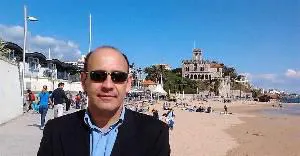# Temperature and Humidity Logger (Using Arduino)

Reads and stores in SD card, the temperature and humidity values over time.

IntermediateShowcase (no instructions)16,724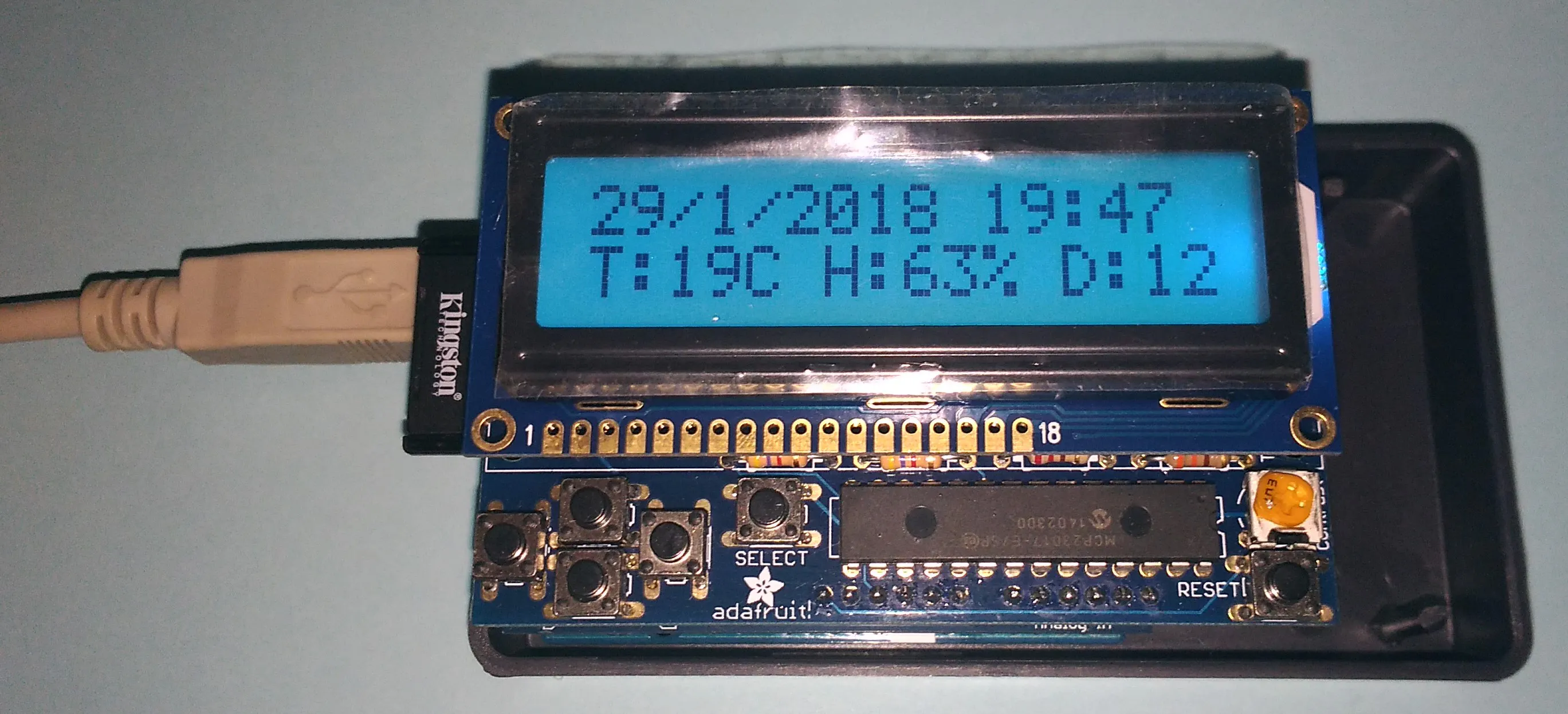## Things used in this project

### Hardware components

 Adafruit Data Logger Shield SD CARD Writer, RTC, plus a prototyping area
×1
 Adafruit LCD Shield Kit w/ 16x2 Character Display - Only 2 pins used! This kit uses only 2 arduino pins (SCL and SDA) saving I/O pins for other purposes.
×1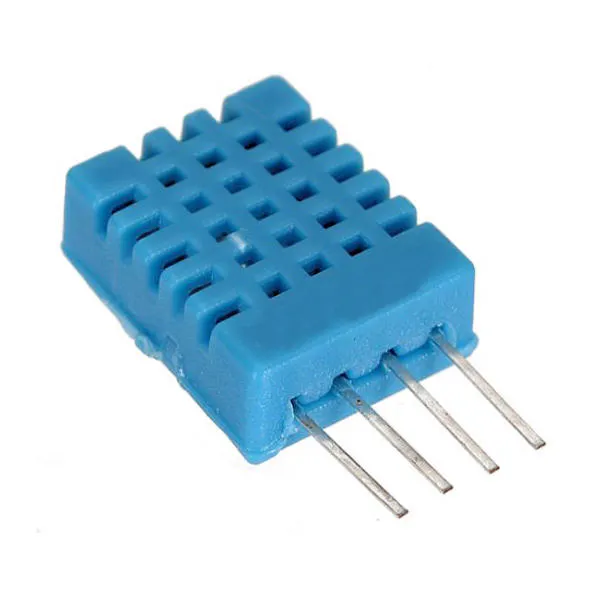DHT11 Temperature & Humidity Sensor (4 pins)
×1Arduino UNO & Genuino UNO I used Arduino UNO R3
×1

### Software apps and online servicesArduino IDE

### Hand tools and fabrication machinesSoldering iron (generic) Medium soldering skills needed

## Schematics

### Temperature and humidity Sensor DHT11 connections

The DHT11 is mounted on the prototyping area of the data logger shield. The diagram is the one showed in this picture.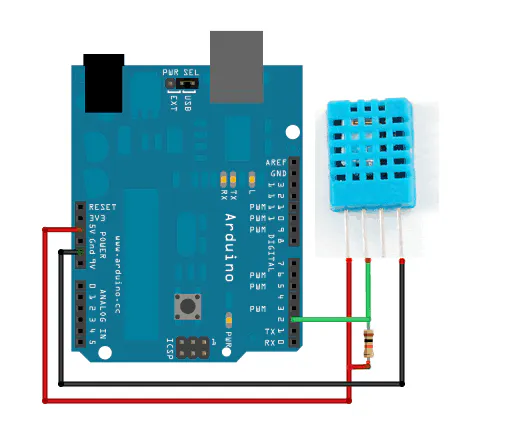### Sensor Diagram

Fritzing Schematic

### Sensor Connection Diagram

jpg file, showing how to connect the sensors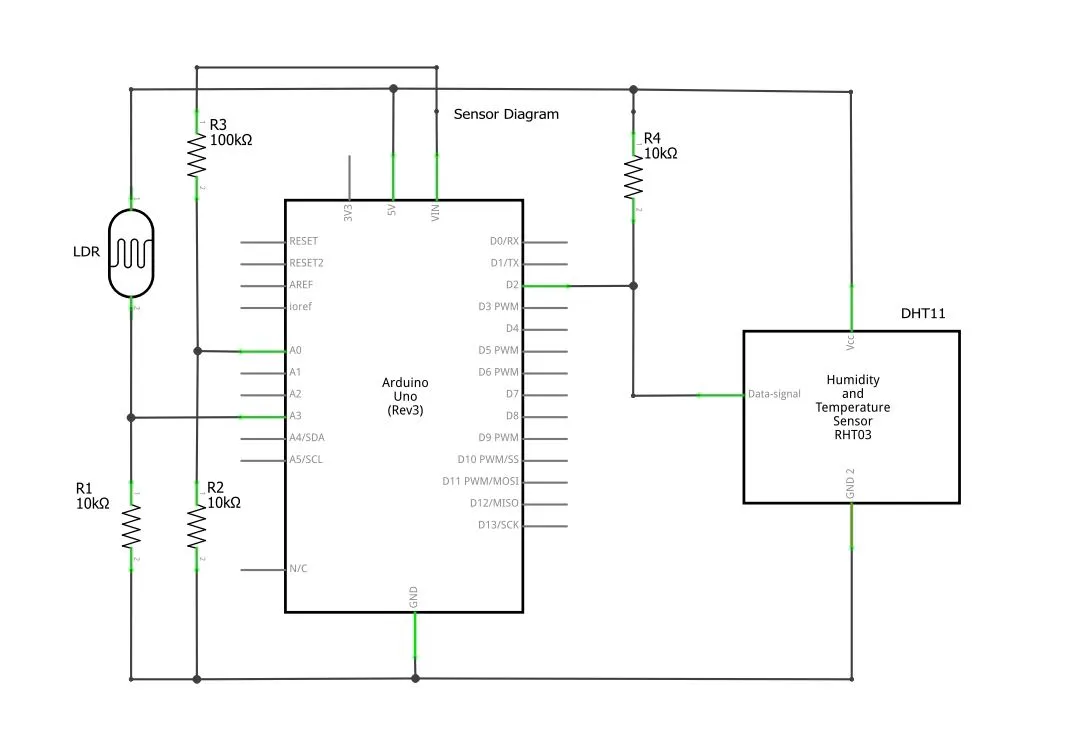### Display connections

4 wires is all you need to connect this display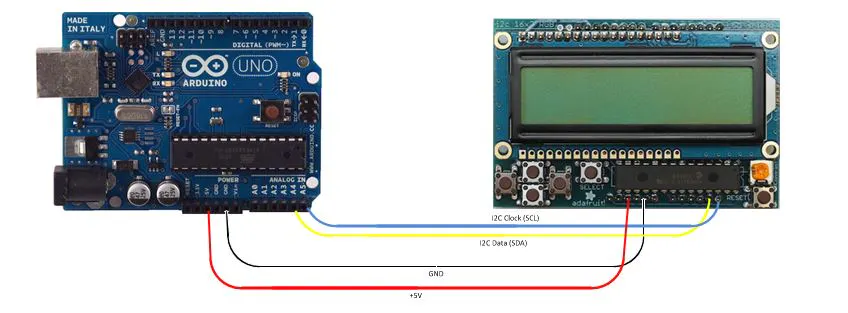## Code

### DataLog_V55.ino

Arduino
This is the final code. Uses Temperature and humidity sensor DHT11.
```/* Arduino Data Logger Version 2015 by Luis Sousa */

#include <SPI.h>
#include <SD.h>
#include <Wire.h>
#include <RTClib.h>
#include <dht11.h>

#define ECHO_TO_SERIAL 1 // dados no porto serie?
#define DHT11PIN 2 //pino digital onde liga sensor de temp e humid
#define redLEDpin 4 //pino digital onde liga LED vermelho
#define greenLEDpin 3 //pino digital onde liga LED verde
#define chipSelect 10 //pino digital onde liga CS do SD Card
#define luzP 3 // pino analogico onde liga LDR
#define pausa 5000 //5s
#define cabecalho "millis,Data,Hora,Vin,Luz,Temperatura,Humidade,DewPoint" //cabecalho da informaao a gravar
#define RED 0x1
#define YELLOW 0x3
#define GREEN 0x2
#define TEAL 0x6
#define BLUE 0x4
#define VIOLET 0x5
#define WHITE 0x7

dht11 DHT11; // sensor de temp e humidade
RTC_DS1307 RTC; // Real Time Clock

// Inicializao de variaveis
float Vin = 0;
byte LCDColor = 1;
int year = 0;
int month = 0;
int day = 0;
int hour = 0;
int minute = 0;
byte buttons = 0;
DateTime now;

//Funoes
void erro(char *str)
{
//Sinaliza erro no lcd
lcd.clear();
lcd.setBacklight(RED);
lcd.setCursor(0, 0);
//lcd.print("Erro ");
lcd.setCursor(0, 1);
lcd.print(str);

//Sinaliza erro no porto serie
Serial.print("Erro: ");
Serial.println(str);
// red LED = erro
digitalWrite(redLEDpin, HIGH);
while (1); // HALT
}
{
byte sair = 0;
byte debounce = 80;
lcd.clear();
lcd.setCursor(0, 0);
lcd.print("Acertar Relogio ");

while (!sair)
{
lcd.setCursor(0, 1);
lcd.print (str);
lcd.print(valor);
if (buttons)
{
if (buttons & BUTTON_UP)
{
valor ++;
delay(debounce);
}
if (buttons & BUTTON_DOWN)
{
valor --;
delay(debounce);
}
if (buttons & BUTTON_LEFT)
{
valor --;
delay(debounce);
}
if (buttons & BUTTON_RIGHT)
{
valor ++;
delay(debounce);
}
if (buttons & BUTTON_SELECT)
{
sair = 1;
}
}
}
return (valor);
}
/*
//Celsius para Fahrenheit
double Fahrenheit(double celsius)
{
return 1.8 * celsius + 32;
}

//Celsius para Kelvin
double Kelvin(double celsius)
{
return celsius + 273.15;
}
*/
// dewPoint function NOAA
// reference: http://wahiduddin.net/calc/density_algorithms.htm
double dewPoint(double celsius, double humidity)
{
double A0 = 373.15 / (273.15 + celsius);
double SUM = -7.90298 * (A0 - 1);
SUM += 5.02808 * log10(A0);
SUM += -1.3816e-7 * (pow(10, (11.344 * (1 - 1 / A0))) - 1) ;
SUM += 8.1328e-3 * (pow(10, (-3.49149 * (A0 - 1))) - 1) ;
SUM += log10(1013.246);
double VP = pow(10, SUM - 3) * humidity;
double T = log(VP / 0.61078); // temp var
return (241.88 * T) / (17.558 - T);
}
/*
// delta max = 0.6544 wrt dewPoint()
// 5x faster than dewPoint()
// reference: http://en.wikipedia.org/wiki/Dew_point
double dewPointFast(double celsius, double humidity)
{
double a = 17.271;
double b = 237.7;
double temp = (a * celsius) / (b + celsius) + log(humidity / 100);
double Td = (b * temp) / (a - temp);
return Td;
}
*/
void setup()
{
pinMode(10, OUTPUT);  // SD ChipSelect
pinMode(13, OUTPUT);  // LED de monitorizaao
pinMode(redLEDpin, OUTPUT);
pinMode(greenLEDpin, OUTPUT);

// Inicializaao do porto serie:
Serial.begin(9600);

//Inicializaao do I2C
Wire.begin();

//Inicializao do LCD
lcd.begin(16, 2);
lcd.setBacklight(TEAL);
lcd.clear();
lcd.setCursor(0, 0);
lcd.print("Data Logger V5.5");
lcd.setCursor(0, 1);
lcd.print("Luis Sousa-2015");
delay(900);
lcd.clear();

//Inicializaao do sensor de temperatura
Serial.print("DHT11 LIBRARY VERSION: ");
Serial.println(DHT11LIB_VERSION);
Serial.println();
Serial.print("Sensor de Temperatura: ");
switch (chk)
{
case 0: Serial.println("OK"); break;
case -1: erro("DHT11 Checksum"); break;
case -2: erro("DHT11 Time out"); break;
default: erro("DHT11 Error"); break;
}
Serial.println();

//Inicializao do SD Card
if (!SD.begin(chipSelect)) // Verifica Presena do SD Card:
{
erro("Introduza SD");
/*
Serial.println("Introduza Cartao SD");
lcd.clear();
lcd.setCursor(0, 0);
lcd.print("Introduza ");
lcd.setCursor(0, 1);
lcd.print("Cartao SD");
// Sai por falta de Cartao
return;
*/
}
else Serial.println("Cartao SD OK.");

// cria novo ficheiro
//char filename[] = "LOGDAT00.CSV";
//for (uint8_t i = 0; i < 100; i++)
//{
// filename = i / 10 + '0';
// filename = i % 10 + '0';
//  if (! SD.exists(filename))
//  {
//    // se nao existir, cria novo
File logfile = SD.open(ficheiro, FILE_WRITE);
//    break; // sai do loop!
//  }
//}
if (! logfile)
{
erro("Erro no ficheiro");
}
else
{
Serial.print("Gravando em: ");
Serial.println(ficheiro);
logfile.println(cabecalho);
logfile.close();
}
#if ECHO_TO_SERIAL
Serial.println(cabecalho);
#endif //ECHO_TO_SERIAL

//Inicializao do RTC
if (!RTC.begin())
{
// logfile.println("RTC com avaria");
// Serial.println("RTC com avaria");
erro("RTC com avaria");
}
if (! RTC.isrunning())
{
// linha seguinte acerta RTC para data/hora da compilaao
// para ajustar para uma determinada data/hora por ex:
// 21 de Janeiro de 2014 as 03h escreveria:
// rtc.adjust(DateTime(2014, 1, 21, 3, 0, 0));
}
}

void loop()
{
digitalWrite(greenLEDpin, HIGH); // Inicia Escrita

Vin = (55.00 * analogRead(0) / 1024);
float humid = DHT11.humidity;
float tempC = DHT11.temperature;
tempC = tempC - 2;  // Correco apos calibrao
//float tempK = Kelvin(tempC);
//float tempF = Fahrenheit(tempC);
float dewP = dewPoint(tempC, humid);
/*
if (Vin < 7.50) lcd.setBacklight(0); //modo poupana de energia
else lcd.setBacklight(TEAL);
*/
DateTime now;
now = RTC.now();
uint32_t m = millis();

//display data
lcd.setCursor(0, 0);
lcd.print(now.day(), DEC);
lcd.print("/");
lcd.print(now.month(), DEC);
lcd.print("/");
lcd.print(now.year(), DEC);
lcd.print(" ");
lcd.print(now.hour(), DEC);
lcd.print(":");
lcd.print(now.minute(), DEC);
lcd.print("  ");
lcd.setCursor(0, 1);
lcd.print("T:");
lcd.print(tempC, 0);
lcd.print("C H:");
lcd.print(humid, 0);
lcd.print("% ");
lcd.print("D:");
lcd.print(dewP, 0);
lcd.print("  ");

// log data
File logfile = SD.open(ficheiro, FILE_WRITE);
if (logfile)
{
logfile.print(m); // millisegundos desde o inicio
logfile.print(",");
logfile.print(now.day(), DEC);
logfile.print("/");
logfile.print(now.month(), DEC);
logfile.print("/");
logfile.print(now.year(), DEC);
logfile.print(",");
logfile.print(now.hour(), DEC);
logfile.print(":");
logfile.print(now.minute(), DEC);
logfile.print(":");
logfile.print(now.second(), DEC);
logfile.print(",");
logfile.print(Vin, 2);
logfile.print(",");
logfile.print(luzV);
logfile.print(",");
logfile.print(tempC, 0);
logfile.print(",");
logfile.print(humid, 0);
logfile.print(",");
logfile.println(dewP, 0);
logfile.close();
}
else
{
erro("Erro no ficheiro");
}
#if ECHO_TO_SERIAL
Serial.print(m); // millisegundos desde o inicio
Serial.print(",");
Serial.print(now.day(), DEC);
Serial.print("/");
Serial.print(now.month(), DEC);
Serial.print("/");
Serial.print(now.year(), DEC);
Serial.print(",");
Serial.print(now.hour(), DEC);
Serial.print(":");
Serial.print(now.minute(), DEC);
Serial.print(":");
Serial.print(now.second(), DEC);
Serial.print(",");
Serial.print(Vin, 2);
Serial.print(",");
Serial.print(luzV);
Serial.print(",");
Serial.print(tempC, 0);
Serial.print(",");
Serial.print(humid, 0);
Serial.print(",");
Serial.println(dewP, 0);
#endif //ECHO_TO_SERIAL

digitalWrite(greenLEDpin, LOW); // acabou escrita

//Teste aos butoes do LCD
for (byte i = 0; i < 20; i++)
{
delay(pausa / 20);
if (buttons)
{
if (buttons & BUTTON_UP)
{
lcd.setCursor(0, 1);
lcd.print("Vin: ");
lcd.print(Vin, 2);
lcd.print("V      ");
}
if (buttons & BUTTON_DOWN)
{
lcd.setCursor(0, 1);
lcd.print("Luz: ");
lcd.print(luzV);
lcd.print("        ");
delay(500); //debounce
if (buttons & BUTTON_DOWN)
{
now = RTC.now();
year = now.year();
month = now.month();
day = now.day();
hour = now.hour();
minute = now.minute();
RTC.adjust(DateTime(year, month, day, hour, minute, 0));
lcd.setCursor(0, 1);
char msg[] = "RTC Ajustado    ";
lcd.print(msg);
logfile.println(msg);
Serial.println(msg);
}
}
if (buttons & BUTTON_LEFT)
{
LCDColor = LCDColor - 1;
if (LCDColor < 1) LCDColor = 1;
lcd.setBacklight(LCDColor);
}
if (buttons & BUTTON_RIGHT)
{
LCDColor = LCDColor + 1;
if (LCDColor > 7) LCDColor = 7;
lcd.setBacklight(LCDColor);
}
if (buttons & BUTTON_SELECT)
{
if (LCDColor  > 0)LCDColor = 0;
else LCDColor = (TEAL);
lcd.setBacklight(LCDColor);
}
}
}
}
```

## Credits

### lmsousa

5 projects • 18 followers